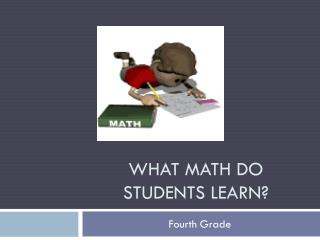Download PresentationWhat Math do students learn?

# What Math do students learn?

Download Presentation## What Math do students learn?

- - - - - - - - - - - - - - - - - - - - - - - - - - - E N D - - - - - - - - - - - - - - - - - - - - - - - - - - -
##### Presentation Transcript

1. What Math do students learn? Fourth Grade

2. Focus on Understanding This year students will be learning the Mathematics Florida Standards (MAFS). One of the most important things about the standards is that students need to DEEPLY understand math concepts.

3. Critical Areas The MAFS have Critical Areas for each grade level, these are the main focus of math instruction. For Fourth Grade, these are: • Developing understanding and fluency with multi-digit multiplication, and developing understanding of dividing to find quotients involving multi-digit dividends • Developing an understanding of fraction equivalence, addition and subtraction of fractions with like denominators, and multiplication of fractions by whole numbers • Understanding that geometric figures can be analyzed and classified based on their properties, such as having parallel sides, perpendicular sides, particular angle measures, and symmetry • Using grade-level appropriate Mathematical Practices

4. This is HOW students will be learning about the content.

5. Report Card The most important standards for Fourth Grade are evaluated on the report card: • *Demonstrates understanding of place value concepts up to 1,000,000 and of decimals • * Fluently adds and subtracts and uses efficient strategies to multiply and divide • * Understands fraction equivalence, adds and subtracts fractions with like denominators, and multiplies a fraction by a whole number • Uses algebraic thinking to determine the unknown in an equation and analyzes patterns • Solves problems involving measurement and converting units • Constructs and analyzes line plots with fractions • * Classifies 2-D shapes by their lines and angles and draws lines of symmetry • * Uses grade-level appropriate mathematical practices *Denotes critical Power Standard for the grade level.

6. Investigations To help build a deep understanding, we will be spending the majority of our time working with Investigations in Number, Data, and Space.

7. Investigations in Class In school, students: • investigate problems • work in collaborative groups • use various strategies • communicate thinking orally and in writing • play educational games

8. Investigations at Home At home, students may be asked to: • practice an activity or game from class • collect some information to be used in class • solve a few problems and explain how they solved the problems • solve problems in more than one way

9. Helping at Home You can help your child by: • reading all of the Family Letters that are sent home • helping your child find a place at home to keep game materials and directions that are sent home • playing the games together that are sent home

10. Helping at Home • asking your child questions when they are stuck rather than giving them an answer • “What do you need to find out?” • “How can you get started?” • “Have you done anything like this in school?” • keeping a positive attitude about math

11. Helping at Home Online Student and Parent access to math software and Student Math Handbook

12. Florida Standards Assessment (FSA) • Students in 4th grade will take the Math FSA at the beginning of Quarter 4. • FSA will test students’ understanding of the MAFS. • The questions on FSA expect students to deeply understand concepts. • http://fsassessments.org/

13. FSA Sample 4th Grade Question

14. Parent Resources Visit the District website’s Elementary Math page for more information about the 4th Grade standards, our math program, and how you can assist your child: http://www.collier.k12.fl.us/math/mcE.htm Visit the Investigations website for games and activities to play online: http://investigations.terc.edu/families/doing_math/games_index/